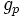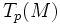# Riemannian manifold

This article defines a differential manifold with the following additional structure -- the structure group is reduced to: orthogonal group

## Definition

A Riemannian manifold is the following data:

1. A differential manifold$M$
2. A Riemannian metric$g$ on$M$, viz a smooth association of a positive definite symmetric bilinear form$g_p$ for the tangent space$T_p(M)$.